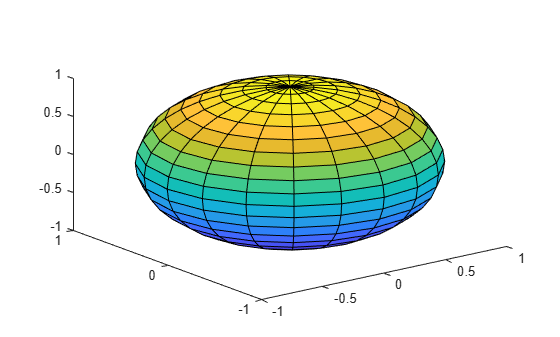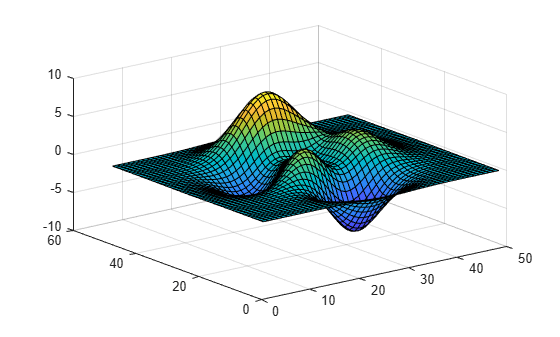# surf2patch

Convert surface data to patch data

## Syntax

```fvc = surf2patch(h) fvc = surf2patch(Z) fvc = surf2patch(Z,C) fvc = surf2patch(X,Y,Z) fvc = surf2patch(X,Y,Z,C) fvc = surf2patch(...,'triangles') [f,v,c] = surf2patch(...) ```

## Description

`fvc = surf2patch(h)` converts the geometry and color data from the `surface` object, `h`, into patch format. The output, `fvc`, is a structure containing the face, vertex, and color data. You can pass this structure directly to the `patch` command.

`fvc = surf2patch(Z) ` calculates the patch data from the surface's `ZData` matrix `Z`.

`fvc = surf2patch(Z,C) ` calculates the patch data from the surface's `ZData` and `CData` matrices `Z` and `C`.

`fvc = surf2patch(X,Y,Z) ` calculates the patch data from the surface's `XData`, `YData`, and `ZData` matrices `X`, `Y`, and `Z`.

`fvc = surf2patch(X,Y,Z,C) ` calculates the patch data from the surface's `XData`, `YData`, `ZData`, and `CData` matrices `X`, `Y`, `Z`, and `C`.

`fvc = surf2patch(...,'triangles') ` creates triangular faces instead of the quadrilaterals that compose surfaces.

`[f,v,c] = surf2patch(...) ` returns the face, vertex, and color data in the three arrays `f`, `v`, and `c` instead of a struct.

## Examples

collapse all

Use the `sphere` command to generate the `XData`, `YData`, and `ZData` of a surface. Then, calculate the patch data. Pass the `ZData` (`z`) to `surf2patch` as both the third and fourth arguments - the third argument is the `ZData` and the fourth argument is taken as the `CData`. You must do this since the `patch` command does not automatically use the z-coordinate data for the color data, as does the surface command.

Since patch is a low-level command, you must set the view and shading to produce the same results produced by the `surf` command.

```[x,y,z] = sphere; figure patch(surf2patch(x,y,z,z)); shading faceted; view(3)```Calculate face, vertex, and color data from a surface whose handle has been passed as an argument.

```figure s = surf(peaks); patch(surf2patch(s)); delete(s) shading faceted; view(3)```